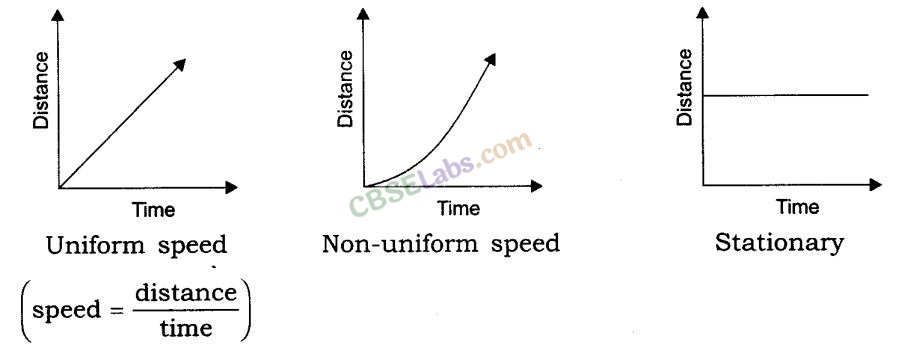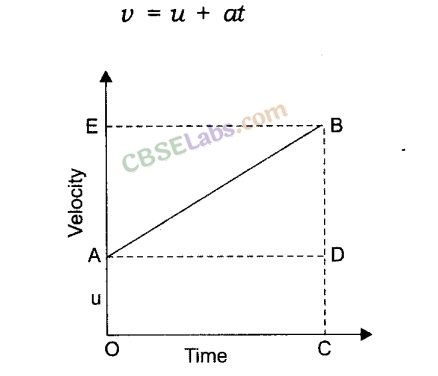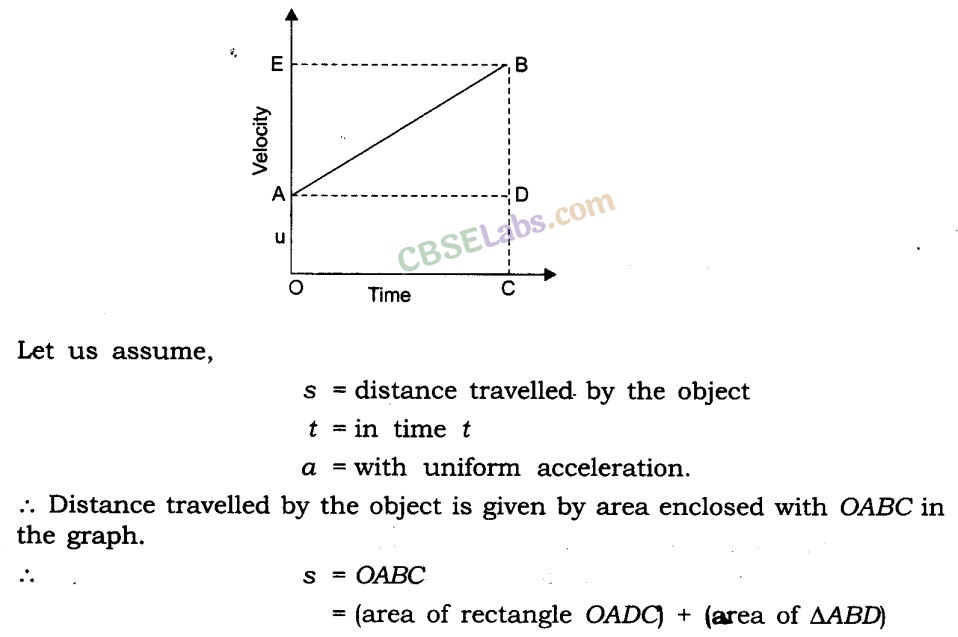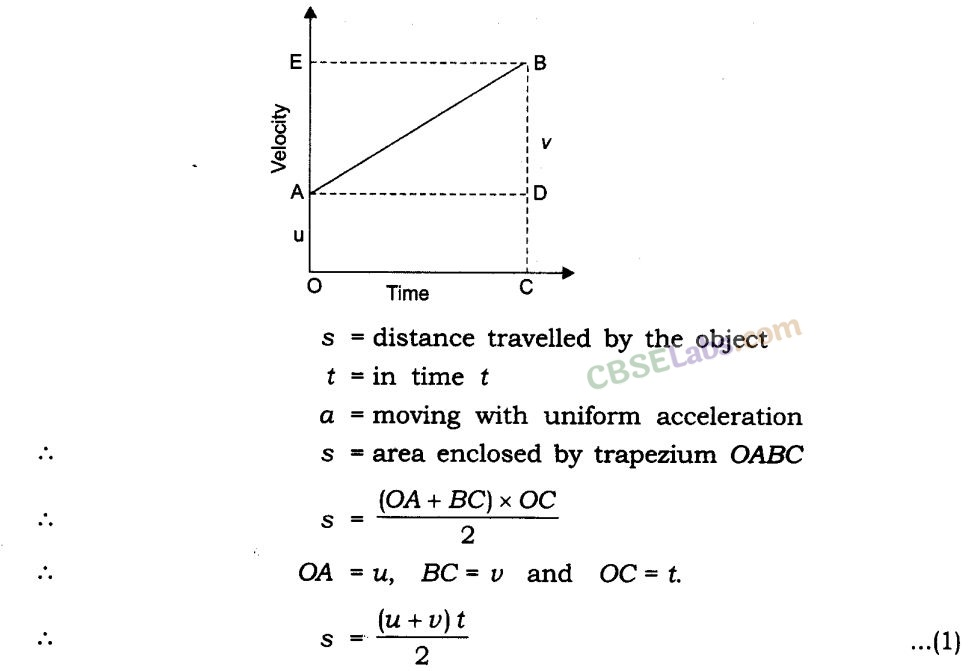Motion Class 9 Notes – Here We have provided summary and revision notes for Class 9 Science Chapter 8. This CBSE notes contains CBSE Key Notes, CBSE Revision Notes, Short Key Notes, images, diagrams of the complete Chapter 8 titled Motion of Science taught in class 9. If you are a student of class 9 who is using NCERT Textbook to study Science, then you must come across Chapter 8 Motion. After you have studied lesson, you must be looking for notes to memorize. Here you can get complete Chapter 8 Motion class 9 notes in one place. For a better understanding of this chapter, you should also see NCERT Solutions for Class 9 Science Chapter 8 Motion.

CBSE Class 9 Science Notes Chapter 8 Motion Pdf free download is part of Class 9 Science Notes for Quick Revision. Here we have given NCERT Class 9 Science Notes Chapter 8 Motion. https://meritbatch.com/motion-cbse-notes-class-9-science/

## CBSE Class 9 Science Notes Chapter 8 Motion

Motion Class 9 Notes
Facts that Matter

An object is said to be in motion when its position changes with time.

We describe the location of an object by specifying a reference point. Motion is relative. The total path covered by an object is said to be the distance travelled by it.

The shortest path/distance measured from the initial to the final position of an object is known as the displacement.

Motion Notes Class 9
Uniform motion:
When an object covers equal distances in equal intervals of time, it is said to be in uniform motion.

Non-uniform motion: Motions where objects cover unequal distances in equal intervals of time.

Speed: The distance travelled by an object in unit time is referred to as speed. Its unit is m/s.

Average speed: For non-uniform motion, the average speed of an object is obtained by dividing the total distance travelled by an object by the total time taken.Velocity: Velocity is the speed of an object moving indefinite direction. S.I. unit is m/s.Acceleration: Change in the velocity of an object per unit time.Graphical representation of motions
(i) Distance-time graph
For a distance-time graph, time is taken on x-axis and distance is taken on the y-axis.
[Note: All independent quantities are taken along the x-axis and dependent quantities are taken along the y-axis.](ii) Velocity-time graphEquation of motion by graphical methods
(i)velocity-time relation:(ii) The equation for position-time relation:(iii) Equation for position-velocity relation:Uniform circular motion: When a body moves in a circular path with uniform speed, its . motion is called uniform circular motion.

We hope the given CBSE Class 9 Science Notes Chapter 8 Motion Pdf free download will help you. If you have any query regarding NCERT Class 9 Science Notes Chapter 8 Motion, drop a comment below and we will get back to you at the earliest.